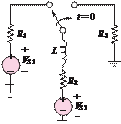### Create an Account

Already have account?

### Forgot Your Password ?

Home / Questions / At t 0 the circuit shown in Figure P529 is at steady state The switch is changed as shown at

# At t 0 the circuit shown in Figure P529 is at steady state The switch is changed as shown at

At 0, the circuit shown in Figure P5.29 is at steady state. The switch is changed as shown at = 0.

VS1  = 13 V         VS2  = 13 V = 170 mH      R1  = 2.R2  = 4.3 kQ    R3  = 29 kQ

Determine the time constant of the circuit for 0changed at = 0 as shown.

VS1  = 17 V         VS2  = 11 V

R1  = 14 kQ       R2  = 13 kQ

R3  = 14 kQ       = 70 nF

Jun 19 2020 View more View Less

#### Answer (Solved)Subscribe To Get Solution